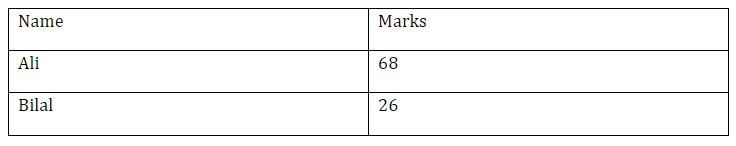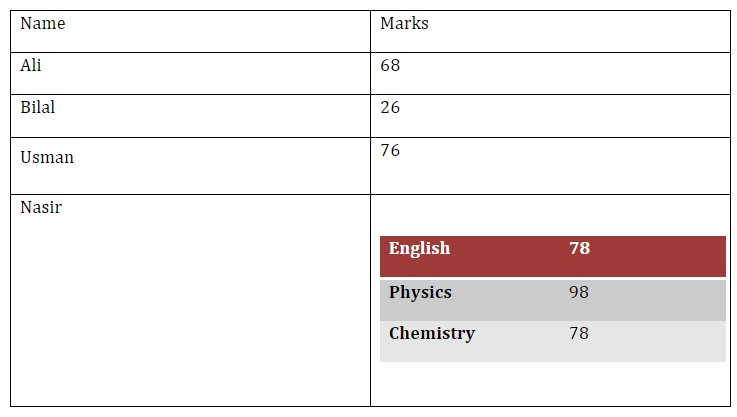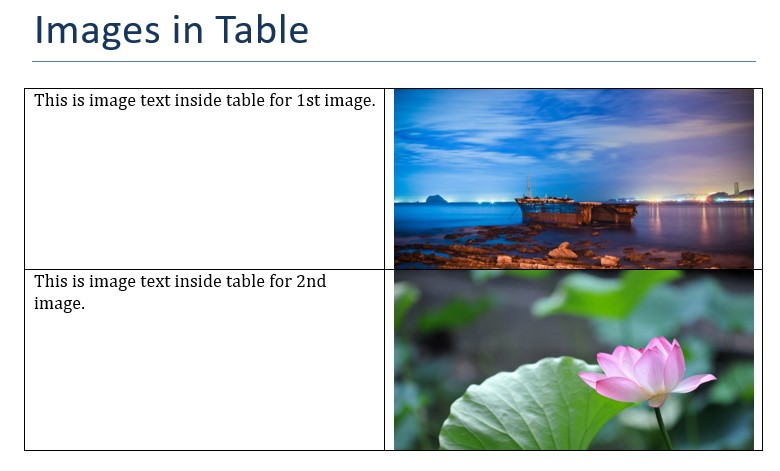Python-docx provides a lot of features for creating and modifying word documents. In this tutorial, we will implement `table` feature and use table rows and columns to add data to tables. For API documentation of tables in python-docx, check documentation on this url.

Tables are constructed using `add_table()` method in python-docx document. We can provide following options while creating a table.

• rows: Number of rows for table
• cols: Number of columns for table
• style:  (default `None`) Specify a style, style may be a paragraph style object or paragraph style name.

For complete list of table styles, view on python-docx.

### Create Table

Lets Create a table with 2 rows and 2 colums using `add_table` method. Table style can be defined using style argument which in this case is Table Grid.

``````from docx import Document
# create document
doc = Document()

table = doc.add_table(rows=2, cols=2, style="Table Grid")``````

Now table rows and columns can be accessed using `table.rows` or `table.columns` attribute respectively and then we can access each cell in that row using `row.cells`. Lets add headings to 1st row.

``````# access first row's cells

Same way we can add other info to rows

``````# access second row's cells
data_row = table.rows.cells

data_row.text = "Ali"
data_row.text = "68"``````

We can also add rows after table creation using add_row method which adds a row in table and we can add data to that row.

``````# add new row to table

data_row.text = "Bilal"
data_row.text = "26"``````

It creates a table with 3 rows and 2 columns with information.### Cell Margin

We can use OpenXML to modify and set any style using python-docx. Here we can specify margin to any cell where we can add or completely remove a cell margin.

``````from docx.oxml.shared import OxmlElement
from docx.oxml.ns import qn

def set_cell_margins(cell, **kwargs):
"""
cell:  actual cell instance you want to modify
usage:
set_cell_margins(cell, top=50, start=50, bottom=50, end=50)

provided values are in twentieths of a point (1/1440 of an inch).
"""
tc = cell._tc
tcMar = OxmlElement('w:tcMar')

for m in ["top", "start", "bottom", "end"]:
if m in kwargs:
node = OxmlElement("w:{}".format(m))
node.set(qn('w:w'), str(kwargs.get(m)))
node.set(qn('w:type'), 'dxa')
tcMar.append(node)

tcPr.append(tcMar)``````

From Stackoverflow: https://stackoverflow.com/a/55177526/6663675

Now we can add margin in any cell to increase space.

``````# access second row's cells

set_cell_margins(data_row, top=100, start=100, bottom=100, end=50)

data_row.text = "Usman"
data_row.text = "76"``````

### Nested Table

We can also created nested tables where we can add a table to a cell. For example, if for 1 person, we want to add marks for multiple subjects. We can add a table to parent table cells.

``````marks = {"English" : 78, "Physics" : 98, "Chemistry" : 78}

# name of person
data_row.text = "Nasir"
# We add a table to 2nd cell with rows equal to our entries (3)

Now we can iterate over values and add to table.

``````for i, row in enumerate(marks.items()): # iterate over 3 values
marks_table.rows[i].cells.text = row # sub table first cell
marks_table.rows[i].cells.text = str(row) # second cell``````We can also show images inside tables.

### Table Images

In table paragraphs, we can add images to table. Here is a simple example to add two images to table. Here we get paragraph for a cell where we want to display image and then use add_picture method to add an image from path. and we also specify height and width in Inches.

``````from docx.shared import Inches, Cm

doc = Document() # create doc

# create table with two rows and columns
table = doc.add_table(rows=2, cols=2, style="Table Grid")

# add first image with text
table.rows.cells.text = 'This is image text inside table for 1st image.'

paragraph = table.rows.cells.paragraphs

table.rows.cells.text = 'This is image text inside table for 2nd image.'

paragraph = table.rows.cells.paragraphs

# save to file
doc.save("images-table.docx")``````

So it creates a table with text in 1st column and images in 2nd column for each row.For more info to working with images, view my next post or view python-docx documentation.

View can add data from csv file, text file or any other file to tables in docx. So for more info view python docx documentation.